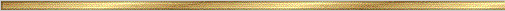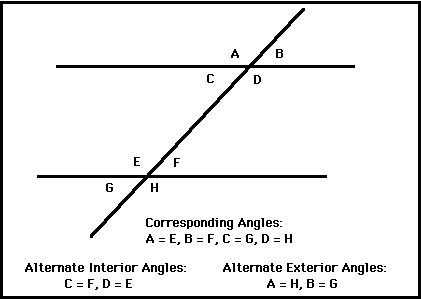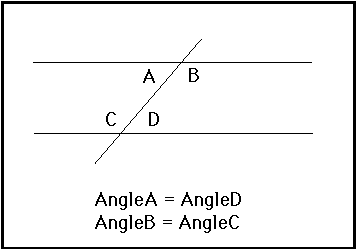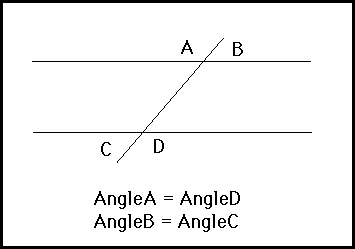# Parallel Lines Conjectures### Explanation:

A line passing through two or more other lines in a plane is called a transversal. A transversal intersecting two parallel lines creates three different types of angle pairs. They are:
• corresponding angles
• alternate interior angles
• alternate exterior angles

### The precise statement of the conjecture is:

Conjecture (Corresponding Angles Conjecture ): If two parallel lines are cut by a transversal, the corresponding angles are congruent.Conjecture (Alternate Interior Angles Conjecture ): If two parallel lines are cut by a transversal, the alternate interior angles are congruent.Conjecture (Alternate Exterior Angles Conjecture ): If two parallel lines are cut by a transversal, the corresponding angles are congruent.• Key Curriculum Press can provide demo versions of Geometer's Sketch Pad